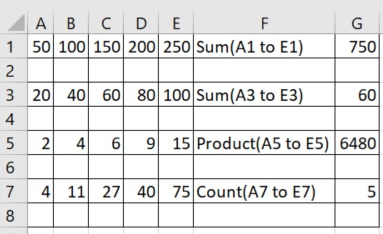# Arithmetic operations in excel file using openpyxl in Python

Python can help us use excel files directly from the python environment. We can refer to the each cell or a range of cells in excel and apply arithmetic operators on those cells. The results of those operations can also be stored at some cells whose location can be specified by the python program.

In the below examples we are performing various arithmetic operations using inbuilt functions of excel. Like sum or average of numbers inside cells. The results are also stored at specific locations. We use the openpyxl module which opens a workbook and marks it active. Then we store certain values in predefined cells and then apply the functions on those cells storing the result in other cells.

## Example

import openpyxl

excel = openpyxl.Workbook()

excel_file = excel.active

excel_file['A1'] = 50
excel_file['B1'] = 100
excel_file['C1'] = 150
excel_file['D1'] = 200
excel_file['E1'] = 250

excel_file['A3'] = 20
excel_file['B3'] = 40
excel_file['C3'] = 60
excel_file['D3'] = 80
excel_file['E3'] = 100

excel_file['A5'] = 2
excel_file['B5'] = 4
excel_file['C5'] = 6
excel_file['D5'] = 9
excel_file['E5'] = 15

excel_file['A7'] = 4
excel_file['B7'] = 11
excel_file['C7'] = 27
excel_file['D7'] = 40
excel_file['E7'] = 75

excel_file['F1'] = 'Sum(A1 to E1)'
excel_file['G1'] = '= SUM(A1:E1)'
excel_file['F3'] = 'Sum(A3 to E3)'
excel_file['G3'] = '= AVERAGE(A3:E3)'
excel_file['F5'] = 'Product(A5 to E5)'
excel_file['G5'] = '= PRODUCT(A5:E5)'
excel_file['F7'] = 'Count(A7 to E7)'
excel_file['G7'] = '= COUNT(A7:E7)'

excel.save("D:\Arithmetic_operations.xlsx")

## Output

Running the above code gives us the following result −